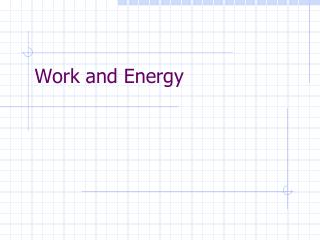Download PresentationWork and EnergyWork and Energy - PowerPoint PPT Presentation

Download PresentationWork and Energy
An Image/Link below is provided (as is) to download presentation

Download Policy: Content on the Website is provided to you AS IS for your information and personal use and may not be sold / licensed / shared on other websites without getting consent from its author. While downloading, if for some reason you are not able to download a presentation, the publisher may have deleted the file from their server.

- - - - - - - - - - - - - - - - - - - - - - - - - - - E N D - - - - - - - - - - - - - - - - - - - - - - - - - - -
Presentation Transcript

1. Work and Energy

2. Work… …is the product of the magnitude of displacement times the component of force parallel to the displacement. W = F‖ d Units: N·m or Joules (J)

3. F  d Work = Fd cos  • Consider a cratepulledacross a surface. Where… • Fistheappliedforce • disthedisplacement • θistheanglebetweentheforce and thedirection F‖ = Fcosθ

4. Consider the same crate being pulled up an incline… Work = Fdcos • θ = ZERO in this situation!!! The force and the displacement are in the same direction F d

5. Work • Net work in moving an object is independent of the path to get there. Same amount of work done More force needed Shorter distance traveled Less force needed Greater distance traveled

6. Energy – What is it? • The ability to do work

7. Types of Energy • Kinetic • Gravitational Potential • Elastic Potential • Heat • Light • Sound • Electrical • Chemical • Nuclear Mechanical Energy – energy associated with an object’s position or motion

8. Consider a cart with an initial velocity vi and a net force acting on it through a distance d… vi vf Fnet Fnet d Wnet = Fnet d Remember F = ma = m(vf2 – vi2/2d) So… Wnet = m(vf2 – vi2/2d)d Wnet = m(vf2 – vi2/2) Wnet = ½ mvf2 – ½ mvi2

9. Energy - Kinetic • Kinetic Energy is the energy that an object possesses due to its motion • If the mass of a body is m and its speed is v then its kinetic energy is given by KE= ½ mv2

10. Work-Energy Theorem • W = ½ mvf2 – ½ mvi2 W = ΔKE • The net work done on an object (by a net force) is equal to a change in kinetic energy of the object

11. Example problem -A 10.0 kg sled is initially moving across a frozen pond at a speed of 4.2 m/s. How far will it travel if the coefficient of friction between the sled and ice is 0.10?

12. Energy - Potential • Potential Energy – the energy of an object due to its position, shape, or condition • An system acquires potential energy when work is done against another force

13. Energy - Potential Gravitational Potential Energy • This is the energy of an object associated with its position in a gravitational field (work done against the force of gravity to put it there) GPE= mgh • m = mass of the object • h = height of object above some fixed position (the position is arbitrary) • g = the acceleration due to gravity

14. Energy - Potential • Elastic Potential Energy • This is the energy that an object possesses due to its position of being stretched or deformed (work done against the elastic restoring force) • FOR A SPRING (or similar)… EPE= ½ k x2 • x = amount of stretch • k = the spring constant (a characteristic of the object being stretched)

15. The Principle of Conservation of Energy • Energy can be transformed from one form to another, but it cannot be created nor destroyed, i.e. the total energy of a system is constant • Energy transformations occur when work is done

16. Conservation of Energy • Since energy is conserved for a system, when we compare the total at two different points… Total E1 = Total E2 KE1 + GPE1 + EPE1 = KE2 + GPE2 + EPE2

17. Power • Power is the rate of doing work or the rate at which energy is transferred. • P = W/t • Units: J/s = Watt • Alternate: P = W/t = Fd/t = Fv